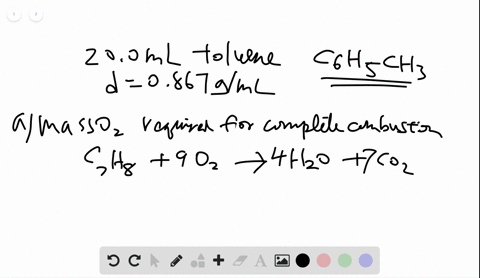🎉 The Study-to-Win Winning Ticket number has been announced! Go to your Tickets dashboard to see if you won! 🎉View Winning Ticket### One of the compounds used to increase the octane …

06:00University of Maine
Problem 106

# The multistep smelting of ferric oxide to form elemental iron occurs at high temperatures in a blast furnace. In the first step, ferric oxide reacts with carbon monoxide to form $\mathrm{Fe}_{3} \mathrm{O}_{4}$ . This substance reacts with more carbon monoxide to form iron(II) oxide, which reacts with still more carbon monoxide to form molten iron. Carbon dioxide is also produced in each step. (a) Write an overall balanced equation for the iron-smelting process. (b) How many grams of carbon monoxide are required to form 45.0 metric tons of iron from ferric oxide?

## Discussion

You must be signed in to discuss.

## Video Transcript

given descriptions of steps of a complex chemical reaction, we can find the overall chemical reaction by adding together the individual equation. The first step is to take our words and write them in equations, So the first reaction has a compound called ferric oxide. Feyerick is the eye on with a plus three charge, and oxide is a to minus. So ferric oxide, the neutral compound is Effie to 03 This reacts with carbon monoxide, which is CEO to make a new compound F E 304 plus carbon dioxide, which is CEO, too. In the second step, take a product that I formed in the first and reacted with more carbon monoxide to make iron two oxide iron to means it has a charge of two plus, and again, oxide is o to minus. So the formula is FV oh, plus carbon dioxide. Finally, the F B o reacts with carbon monoxide to form molten iron, which is just solid metal iron plus sio two. Once we have the skeleton equations, we can balance them for the first equation. I have two irons here and three irons here, so I need a three here and two here for my coefficient. This gives me six irons and six irons. I have a total of 10 oxygen's on the side and 10 oxygen's on this side and one carbon. So the equations balanced for the second equation. Normally, I would balance it looking for the lowest whole number ratio, but in this case, I have to of these produced. So I have to balance the equation, taking that into account so it won't necessarily be the lowest whole number ratio. And in fact, the ratio is two of these with six irons here, for a total of 10 oxygen's on this side in 10 oxygen's on this side and two carbons and to carpet. And so similarly for the last equation, even though the way it's written, it's already balanced. Because I produced six of these. I need six of these to produce 66 so the first step is to find the individual equations. We can then add them and combine like terms to come up with a simplified overall equation for the process. So I have three F E 20 threes, combining with a total of one plus two plus six or nine carbon monoxide plus two f e 304 plus six f e O. Making on my product side to F E 304 was a total of nine carbon dioxides plus six f e o plus six f e, and we can cancel out any terms that appear on both sides. So, for example, there are two F e 30 fours. There are six F EOS that gives us our overall equation of three F e 203 which is a solid plus nine CEO, which is a gas makes nine carbon dioxides, the gas plus six F E solids. It is now appropriate to simplify this. So dividing by the lowest common multiple, which is three. This is a one with three, the three and a two for the overall balanced equation. Using the balanced equation, we can then make conversions if we want to produce, for example, 45 metric tons. So iron. The question is how much how many grams of carbon monoxide are needed. So we need to change our metric tons, two grams our grams of iron, two moles of iron. And we do that using the molar mass once we have moles of iron. We confined moles of carbon monoxide using the balanced equation, the mole ratio. And finally, we can change it to grams of carbon monoxide using the molar mass of carbon monoxide. One metric ton is equal to 1000 kilograms. The Moeller massive iron we find on the periodic table and that's 55 went 845 grams and the molar mass of carbon monoxide we find by adding the massive carbon, which is 12.11 plus the mass of oxygen, 15.999 or 28 0.1 grams. So now we can do our conversion. 45 metrics Chun's times 1000 kilograms for one metric ton and we know there are 1000 grams and every kilogram. Then we changed two moles by dividing by the molar math. And then we can change two moles of CEO using the balanced equation. There are three moles of carbon monoxide for every two moles of iron. And then finally we multiply by the molar mass of carbon monoxide, and this indicates that we should have three 0.39 times 10 to the seven grams CEO is needed